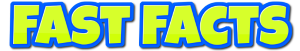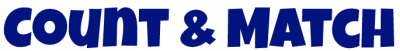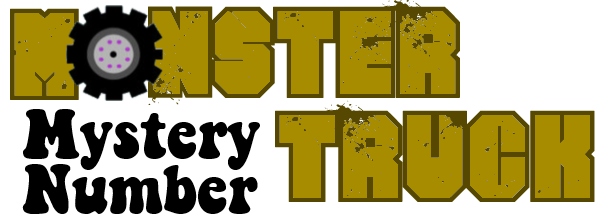Addends(Missing numbers in addition and subtraction.)Basic Facts(Missing numbers in addition, subtraction, multiplication, division, and two-step math.)Basic Facts(Addition, subtraction, multiplication, division)Basic Facts(Addition, subtraction, multiplication, division)Basic Facts & Integers(Addition, subtraction, multiplication, division)Basic Facts(Addition, subtraction, multiplication, division)Basic Facts(Addition, subtraction, multiplication, division)Basic Facts(Addition, subtraction, multiplication, division)Basic Facts(Addition, subtraction, multiplication, division)Comparing Numbers(Greater than or less than)Counting to 10Counting to 10DecimalsFact FamiliesFractionsFractions(Reducing)Money(Counting Coins)MultiplesMultiplicationNumber SenseNumber PatternsPatterns & LogicPlace ValuePlace ValueRounding & EstimatingTime(Reading and elapsed time.)